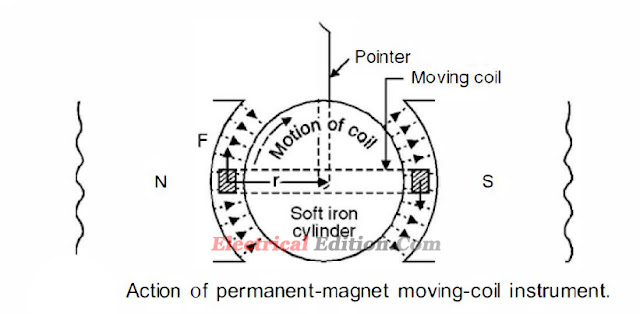Permanent Magnet Moving Coil Type (PMMC) Instrument-Working Principle

Permanent Magnet Moving Coil Type (PMMC) Instrument Working Principle

The basic principle of operation is that when a current carrying conductor is brought in a magnetic field (they should not be parallel to each other) a torque on the conductor is produced. The Permanent Magnet Moving Coil instrument consists of a rectangular coil pivoted so that its sides lie in the air gap between the two poles of a permanent magnet and a soft-iron cylinder. The air gap between the magnet poles and iron core is small and the flux density is uniform and is in a radial direction, so that the flux lines are always at right angle to the current carrying conductor and hence when current passes through the coil, a deflecting torque is produced owing to the interaction between the two fluxes, one due to permanent magnet and the other due to the magnetic field of the coil. This is shown in Fig.1.

constructions of permanent magnet moving coil instrumentsFig.1.
If I is the current flowing in the moving coil in the direction shown, forces F, F will act on the two sides of this coil, the direction of force being determined by Fleming's left-hand rule and is in the direction for this system as shown in Fig.1. The torque causing the coil to rotate is given as F.2r where r is the mean distance of the wires forming the sides of the coil, from the axis of rotation.Now, if N is the no. of turns in the coil, B the magnetic flux density due to permanent magnet, I the current in the coil, l the effective length, the force acting on the coil is given by

F = NBil Newton .. . (4.1)
Hence the torque on the coil is
T = NBil. 2r N-m ... (4.2)

Control torque & Damping torque in  PMMC Instrument

In any measuring (electromagnetic) instrument there are three torques acting on the moving mechanism to which a pointer is connected which moves on the dial of the instrument and indicates the reading of the quantity being measured. One of the three torques is the deflecting torque which we have just studied. The other torques are (i) control torque (ii) Damping torque. In case of PMMC instruments spring made of phosphor bronze provides control torque. The spring also serves as leads to the moving coil. When deflecting torque acts on the coil, both the control torque and damping torques come into action. The control torque restrains the rotation of the coil whereas the deflecting torque tries to rotate the coil. At balance, if the coil has moved through an angle  θ and if k is the spring constant, we have

as NEZ.2r are constant of a particular meter. Hence equation (4.5) suggests that the current is proportional to θ. Thus the scale is uniformly divided i.e. it is a linear scale i.e. if for I amp the deflection is through an angle θ, for 2I amps the deflection would be 2θ.Now if damping torque were absent, the pointer will keep on oscillating around the mean value and an exact reading cannot be recorded. These oscillations are damped out by the damping torque. The moving coil is wound on an aluminium former which is placed in the magnetic field.As the coil rotates eddy currents are induced in the aluminium former and these eddy currents would try to oppose the cause i.e. the deflecting force and finally when the pointer comes to its actual value being measured, damping is provided by eddy currents and the coil (the pointer) remains stationary at its actual value.

Torque Equation of PMMC Instrument

The equation for the developed torque can be obtained from the basic low of the electromagnetic torque. The deflecting torque is given by,

Td   = NBAI
where  Td  = deflecting torque in N-m
B = flux density in air gap, Wb/m2
N = number of turns of the coil
A = Effective coil area m2
I = Current in the moving coil, amperes
Td  = GI
where G = NBA = constant
The controlling torque is provided by the springs and is proportional to the angular deflection of the pointer.
Tc  = kθ
where  Tc  = controlling torque
K = spring constant, Nm/rad or Nm/deg
θ = angular deflection
for the final steady state position,
Td   = Tc
GI = Kθ
θ = (G/K) I
I = (K/G)θ

Note: Thus the deflection is directly proportional to the current passing through the coil in Permanent Magnet Moving Coil Type (PMMC) Instrument.

Advantages of Permanent Magnet Moving Coil Type  or PMMC instruments

(a) Low power consumption
(b) High Torque/weight-ratio
(c) Uniformity of the scale and the possibility of a very long scale
(d) Perfect damping provided by eddy currents induced in the metal former of the moving coil. The metal used is aluminium as it is light in weight
(e) The possibility of a single instrument being used with shunt and resistance to cover a large range of both currents and voltages.
(f) Freedom from errors due to stray magnetic fields.

Tags:PMMC Torque Equation,PMMC instrument wikipedia,permanent magnet moving coil (PMMC),permanent magnet moving coil  (PMMC) mechanism,Working operation